# AC Power

## AC Electric Circuits

• #### Question 1

If a sinusoidal voltage is applied to an impedance with a phase angle of 0o, the resulting voltage and current waveforms will look like this: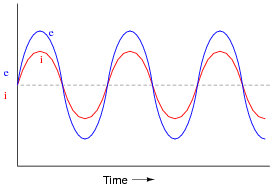Given that power is the product of voltage and current (p = i e), plot the waveform for power in this circuit.

• #### Question 2

If a sinusoidal voltage is applied to an impedance with a phase angle of 90o, the resulting voltage and current waveforms will look like this: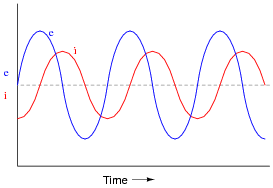Given that power is the product of voltage and current (p = i e), plot the waveform for power in this circuit. Also, explain how the mnemonic phrase ËLI the ICE man” applies to these waveforms.

• #### Question 3

If a sinusoidal voltage is applied to an impedance with a phase angle of -90o, the resulting voltage and current waveforms will look like this: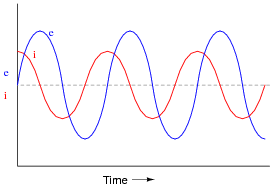Given that power is the product of voltage and current (p = i e), plot the waveform for power in this circuit. Also, explain how the mnemonic phrase ËLI the ICE man” applies to these waveforms.

• #### Question 4

Power is easy to calculate in DC circuits. Take for example this DC light bulb circuit:Calculate the power dissipation in this circuit, and describe the transfer of energy from source to load: where does the energy come from and where does it go to?

• #### Question 5

A generator is coupled to a bicycle mechanism, so that a person can generate their own electricity: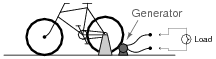The person pedaling this bicycle/generator notices that it becomes more difficult to pedal when the generator is connected to a load such as a light bulb, or when it is charging a battery. When the generator is open-circuited, however, it is very easy to spin. Explain why this is, in terms of work and energy transfer.

• #### Question 6

If the power waveform is plotted for a resistive AC circuit, it will look like this: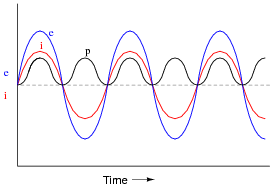What is the significance of the power value always being positive (above the zero line) and never negative (below the zero line)?

• #### Question 7

If the power waveform is plotted for an AC circuit with a 90 degree phase shift between voltage and current, it will look something like this: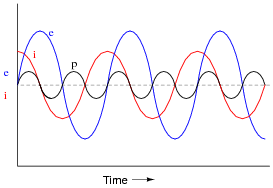What is the significance of the power value oscillating equally between positive (above the zero line) and negative (below the zero line)? How does this differ from a scenario where there is zero phase shift between voltage and current?

• #### Question 8

If this circuit is built and operated, it will be found that the resistor becomes much hotter than the inductor, even though both components drop the exact same amount of voltage and carry the exact same amount of current: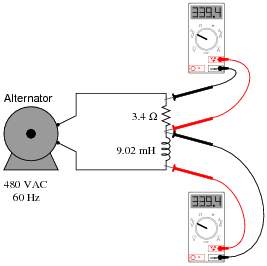Explain why there is such a remarkable difference in heat output between these two components, given their identical voltage drops and currents.

• #### Question 9

Calculate the current in this circuit, and also the amount of mechanical power (in units of “horsepower”) required to turn this alternator (assume 100% efficiency):• #### Question 10

Calculate the current in this circuit, and also the amount of mechanical power (in units of “horsepower”) required to turn this alternator (assume 100% efficiency):• #### Question 11

A student is pondering the behavior of a simple series RC circuit: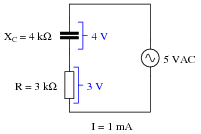It is clear by now that the 4 kΩ capacitive reactance does not directly add to the 3 kΩ resistance to make 7 kΩ total. Instead, the addition of impedances is vectorial:

 √ XC2 + R2 = Ztotal

 ZC + ZR = Ztotal

 (4kΩ ∠ - 90o) + (3kΩ ∠ 0o) = (5kΩ ∠ - 53.13o)

It is also clear to this student that the component voltage drops form a vectorial sum as well, so that 4 volts dropped across the capacitor in series with 3 volts dropped across the resistor really does add up to 5 volts total source voltage:

 VC + VR = Vtotal

 (4V ∠ - 90o) + (3V ∠ 0o) = (5V ∠ - 53.13o)

What surprises the student, though, is power. In calculating power for each component, the student arrives at 4 mW for the capacitor (4 volts times 1 milliamp) and 3 mW for the resistor (3 volts times 1 milliamp), but only 5 mW for the total circuit power (5 volts times 1 milliamp). In DC circuits, component power dissipations always added, no matter how strangely their voltages and currents might be related. The student honestly expected the total power to be 7 mW, but that doesn’t make sense with 5 volts total voltage and 1 mA total current.

Then it occurs to the student that power might add vectorially just like impedances and voltage drops. In fact, this seems to be the only way the numbers make any sense: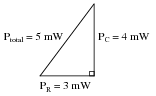However, after plotting this triangle the student is once again beset with doubt. According to the Law of Energy Conservation, total power in must equal total power out. If the source is inputting 5 mW of power total to this circuit, there should be no possible way that the resistor is dissipating 3 mW and the capacitor is dissipating 4 mW. That would constitute more energy leaving the circuit than what is entering!

What is wrong with this student’s power triangle diagram? How may we make sense of the figures obtained by multiplying voltage by current for each component, and for the total circuit?

• #### Question 12

Calculate the current in this circuit, and also the amount of mechanical power (in units of “horsepower”) required to turn this alternator (assume 100% efficiency):• #### Question 13

In this circuit, three common AC loads are modeled as resistances, combined with reactive components in two out of the three cases. Calculate the amount of current registered by each ammeter, and also the amount of power dissipated by each of the loads: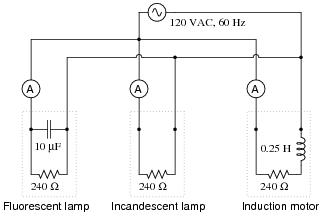If someone were to read each of the ammeters’ indications and multiply the respective currents by the figure of 120 volts, would the resulting power figures (P = I E) agree with the actual power dissipations? Explain why or why not, for each load.

• #### Question 14

A very important parameter in AC power circuits is power factor. Explain what “power factor” is, and define its numerical range.

• #### Question 15

Define true power, in contrast to “reactive” or “apparent” power.

• #### Question 16

Define apparent power, in contrast to “true” or “reactive” power.

• #### Question 17

Define reactive power, in contrast to “true” or “apparent” power.

• #### Question 18

The three different types of power in AC circuits are as follows:

S = apparent power, measured in Volt-Amps (VA)
P = true power, measured in Watts (W)
Q = reactive power, measured in Volt-Amps reactive (VAR)

Explain the names of each of these power types. Why are they called “apparent,” “true,” and “reactive”?

• #### Question 19

Power calculation in DC circuits is simple. There are three formulae that may be used to calculate power:

$$P = IV \ \ \ \ \ \ \ \ \ \ \ P=I^2R \ \ \ \ \ \ \ \ \ \ \ P = \frac{V^2}{R} \ \ \ \ \ Power \ in \ DC \ Circuits$$

Calculating power in AC circuits is much more complex, because there are three different types of power: apparent power (S), true power (P), and reactive power (Q). Write equations for calculating each of these types of power in an AC circuit:

• #### Question 20

Calculate the power factor of this circuit:• #### Question 21

Explain the difference between a leading power factor and a lagging power factor.

• #### Question 22

In this circuit, three common AC loads are represented as resistances, combined with reactive components in two out of the three cases. Calculate the amount of true power (P), apparent power (S), reactive power (Q), and power factor (PF) for each of the loads: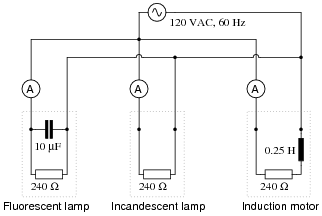Also, draw power triangle diagrams for each circuit, showing how the true, apparent, and reactive powers trigonometrically relate.

• #### Question 23

Calculate the amount of phase shift between voltage and current in an AC circuit with a power factor of 0.97 (lagging), and an apparent power of 3.5 kVA. Also, write the equation solving for phase shift, in degrees.

• #### Question 24

A common analogy used to describe the different types of power in AC circuits is a mug of beer that also contains foam: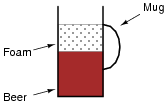Explain this analogy, relating the quantities of beer and foam to the different types of power in an AC circuit, and also why this analogy is often employed to describe the “desirability” of each power type in a circuit.

• #### Question 25

If an electrical device is modeled by fixed values of resistance, inductance, and/or capacitance, it is not difficult to calculate its power factor: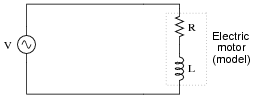P.F. = R

 √ R2 + (ωL)2

In real life, though, things are not so simple. An electric motor will not come labeled with an ideal-component model expressed in terms of R and L. In fact, that would be impossible, as the resistance R in the circuit model represents the sum total of mechanical work being done by the motor in addition to the energy losses. These variables change depending on how heavily loaded the motor is, meaning that the motor’s power factor will also change with mechanical loading.

However, it may be very important to calculate power factor for electrical loads such as multi-thousand horsepower electric motors. How is this possible to do when we do not know the equivalent circuit configuration or values for such a load? In other words, how do we determine the power factor of a real electrical device as it operates?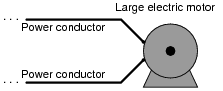Of course, there do exist special meters to measure true power (wattmeters) and reactive power (“var” meters), as well as power factor directly. Unfortunately, these instruments may not be readily available for our use. What we need is a way to measure power factor using nothing more than standard electrical/electronic test equipment such as multimeters and oscilloscopes. How may we do this?

Hint: remember that the angle Θ of the S-Q-P “power triangle” is the same as the angle in a circuit’s Z-X-R impedance triangle, and also the same as the phase shift angle between total voltage and total current.

• #### Question 26

Suppose that a single-phase AC electric motor is performing mechanical work at a rate of 45 horsepower. This equates to 33.57 kW of power, given the equivalence of watts to horsepower (1 HP ≈ 746 W).

Calculate the amount of line current necessary to power this motor if the line voltage is 460 volts, assuming 100% motor efficiency and a power factor of 1.

Now re-calculate the necessary line current for this motor if its power factor drops to 0.65. Assume the same efficiency (100%) and the same amount of mechanical power (45 HP).

What do these calculations indicate about the importance of maintaining a high power factor value in an AC circuit?

• #### Question 27

An oscilloscope is connected to a low-current AC motor circuit to measure both voltage and current, and plot them against one another as a Lissajous figure: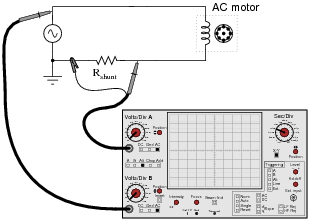The following Lissajous figure is obtained from this measurement: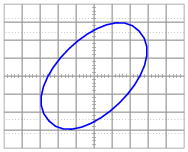From this figure, calculate the phase angle (Θ) and the power factor for this motor circuit.

• #### Question 28

A very high-power AC electric motor needs to have its power factor measured. You and an electrician are asked to perform this measurement using an oscilloscope. The electrician understands what must be done to measure voltage and current in this dangerous circuit, and you understand how to interpret the oscilloscope’s image to calculate power factor.

It would be impractical to directly measure voltage and current, seeing as how the voltage is 4160 volts AC and the current is in excess of 200 amps. Fortunately, PT (“potential transformer”) and CT (“current transformer”) units are already installed in the motor circuit to facilitate measurements: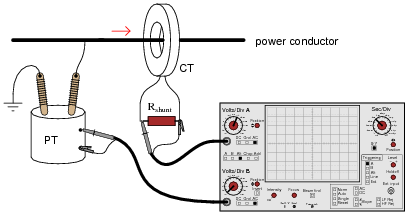After the electrician helps you safely connect to the PT and CT units, you obtain a Lissajous figure that looks like this: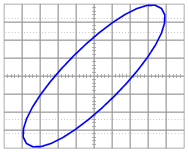Calculate the power factor of the AC motor from this oscilloscope display.

• #### Question 29

A large electrical load is outfitted with a wattmeter to measure its true power. If the load voltage is 7.2 kV and the load current is 24 amps, calculate the load’s apparent power (S). Calculate the power factor and also the phase angle between voltage and current in the circuit if the wattmeter registers 155 kW at those same voltage and current values.

Draw a “power triangle” for this circuit, graphically showing the relationships between apparent power, true power, and phase angle.

• #### Question 30

The power factor of this circuit is as low as it can possibly be, 0: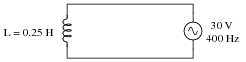Calculate the apparent, true, and reactive power for this circuit:

S =
P =
Q =

Now, suppose a capacitor is added in parallel with the inductor:Re-calculate the apparent, true, and reactive power for this circuit with the capacitor connected:

S =
P =
Q =
• #### Question 31

Calculate the line current and power factor in this AC power system:Now, calculate the line current and power factor for the same circuit after the addition of a capacitor in parallel with the load: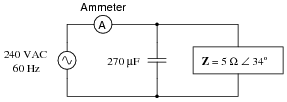• #### Question 32

It is in the best interest of power distribution systems to maintain the power factors of distant loads as close to unity (1) as possible. Explain why.

• #### Question 33

The “power triangle” is a very useful model for understanding the mathematical relationship between apparent power (S), true power (P), and reactive power (Q):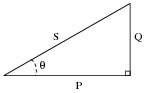Explain what happens to the triangle if power factor correction components are added to a circuit. What side(s) change length on the triangle, and what happens to the angle Θ?

• #### Question 34

When a capacitor is to be connected in parallel with an inductive AC load to correct for lagging power factor, it is important to be able to calculate the reactive power of the capacitor (QC). Write at least one equation for calculating the reactive power of a capacitor (in VARs) given the capacitor’s reactance (XC) at the line frequency.

• #### Question 35

An inductive AC load draws 13.4 amps of current at a voltage of 208 volts. The phase shift between line voltage and line current is measured with an oscilloscope, and determined to be 23o. Calculate the following:

Apparent power (S) =
True power (P) =
Reactive power (Q) =
Power factor =

An electrician suggests to you that the lagging power factor may be corrected by connecting a capacitor in parallel with this load. If the capacitor is sized just right, it will exactly offset the reactive power of the inductive load, resulting in zero total reactive power and a power factor of unity (1). Calculate the size of the necessary capacitor in Farads, assuming a line frequency of 60 Hz.

• #### Question 36

An AC load exhibits a lagging power factor of 0.73 at 230 VAC and 315 amps. If the system frequency is 60 Hz, calculate the following:

Apparent power (S) =
True power (P) =
Reactive power (Q) =
Θ =
Necessary parallel C size to correct power factor to unity =
• #### Question 37

An inductive AC load consumes 15.2 MW of true power at a voltage of 115 kV and 149.8 amps. If the system frequency is 50 Hz, calculate the following:

Apparent power (S) =
Reactive power (Q) =
Power factor =
Θ =
Necessary parallel C size to correct power factor to unity =
• #### Question 38

A dual-trace oscilloscope is used to measure the phase shift between voltage and current for an inductive AC load: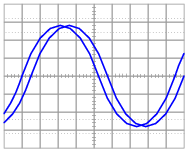Calculate the following, given a load voltage of 110 volts, a load current of 3.2 amps, and a frequency of 60 Hz:

Apparent power (S) =
True power (P) =
Reactive power (Q) =
Θ =
Power factor =
Necessary parallel C size to correct power factor to unity =
• #### Question 39

Calculate the power factor of this circuit: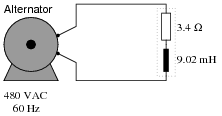Then, calculate the size of the capacitor necessary to “correct” the power factor to a value of 1.0, showing the best location of the capacitor in the circuit.

• #### Question 40

If an AC circuit has a lagging power factor, the way to correct for that power factor is to add a capacitor to the circuit to create leading reactive power. This leading reactive power will cancel the lagging reactive power of the load, ideally negating one another so that there is no reactive power demands placed on the source (zero net reactive power in the circuit):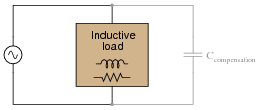Define a step-by-step procedure for calculating the size of compensating capacitor needed (in Farads) in order to correct any lagging power factor to a value of unity. Make your procedure general enough that it may apply to any scenario.

• #### Question 41

Most methods of power factor correction involve the connection of a parallel capacitance to an inductive load:It is technically possible to correct for lagging power factor by connecting a capacitor in series with an inductive load as well, but this is rarely done: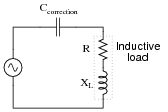Explain why series capacitance is not considered a practical solution for power factor correction in most applications.

• #### Question 42

Although most high-power AC loads are inductive in nature, some are capacitive. Explain what you would have to do to correct for the leading power factor of a large capacitive load, provided the power factor were low enough to warrant the expense of equipment to correct it.

• #### Question 43

In AC power systems, a common way of thinking about reactive power among engineers is in terms of production and consumption. Inductive loads, it is said, consume reactive power. Conversely, capacitive loads produce reactive power.

Explain how the models of “production” and “consumption” relate to reactive power in capacitors and inductors, respectively. Being that neither type of component actually dissipates or generates electrical energy, how can these terms be appropriate in describing their behavior?

• #### Question 44

Another name for “capacitor” is condenser. Explain what a synchronous condenser is, and how it is used to correct power factor in AC power systems.

• #### Question 45

Calculating apparent power for a single-phase AC circuit is easy - simply multiply line voltage by line current (S = VI):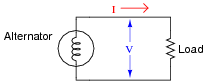How do we calculate apparent power in a balanced three-phase circuit, given the same figures?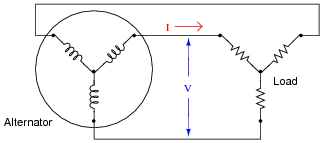• #### Question 46

A very large 3-phase alternator at a hydroelectric dam has the following continuous full-power ratings:

600 MW power output
15 kV line voltage
23.686 kA line current

Calculate the continuous full-load apparent power for this alternator (in MVA), its continuous full-load reactive power (in MVAR), and its power factor (in percent).

• #### Question 47

Large power distribution centers are often equipped with capacitors to correct for lagging (inductive) power factor of many industrial loads. There is never any one value for capacitance that will precisely correct for the power factor, though, because load conditions constantly change. At first it may seem that a variable capacitor would be the answer (adjustable to compensate for any value of lagging power factor), but variable capacitors with the ratings necessary for power line compensation would be prohibitively large and expensive.

One solution to this problem of variable capacitance uses a set of electromechanical relays with fixed-value capacitors:Explain how a circuit such as this provides a step-variable capacitance, and determine the range of capacitance it can provide.